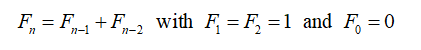# Some Applications of the Fibonacci Numbers

• Yaritza Nunez
• Mulatu Lemma Professor
Keywords: Fibonacci numbers, Fibonacci sequences, Pascal’s triangle

### Abstract

An example of the power of math can be found in Fibonacci numbers.  The Fibonacci numbers are sequences of numbers of the form: 0,1,1,2,3,5,8,13,...  These numbers are famous for possessing wonderful and amazing applications. The sequence plays a central role in elementary number theory. In mathematical terms, it is defined by the following recurrence relation:The first number of the sequence is 0, the second number is 1, and each subsequent number is equal to the sum of the previous two numbers of the sequence itself. That is, after two starting values, each number is the sum of the two preceding numbers. The  Fibonacci numbers appear in an amazing variety of creations, both natural and people-made. The numbers have very interesting properties and keep popping up in many places in nature and art In this paper. Fibonacci sequence also makes its appearance in many different ways within mathematics. In this paper, we investigate some important applications of the Fibonacci numbers.

### Author Biographies

Yaritza Nunez

Department of Mathematics, Savannah State University, USA

Mulatu Lemma, Professor

Department of Mathematics

College of Science and Technology

Savannah State University USA

### References

1. Morris S J, “Fibonacci Numbers”.
http://jwilson.coe.uga.edu/emt669/Student.Folders/Morris.Stephanie/EMT.669/Essay.3/Fibonacci.Essay.html
2.Knott R,Quinney D A and PASS Maths, ,”The life and numbers of Fibonacci”, 1997
http://en.wikipedia.org/wiki/Fibonacci_numbers

4. “Fibonacci Number”. Wolfram MathWorld.the web’s most extensive mathematics resource
http://mathworld.wolfram.com/FibonacciNumber.html

5. Spencer D, Invitation To The Number Theory With Pascals.

6. Burton, D. M., Elementary number theory. New York City, New York: McGraw-Hill. 1998.

7. Koshy T., Fibonacci and Lucas Numbers with Applications, New York City, New York: John Wiley and Sons, Inc. 2001.
Published
2021-07-03
How to Cite
Nunez, Y., & Lemma, M. (2021). Some Applications of the Fibonacci Numbers. GPH - International Journal of Mathematics, 4(06), 38-42. Retrieved from http://www.gphjournal.org/index.php/m/article/view/444
Section
Articles

1 2 > >>